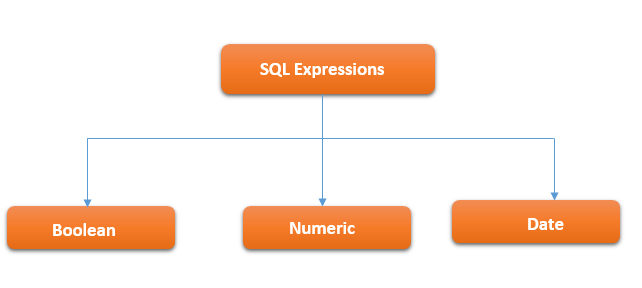# SQL Expressions

SQL Expressions are combination of one or more values, operators and SQL functions that evaluate to a value. These SQL EXPRESSIONs are similar to a formula and they are written in query language. You can also use them to query the database for a specific set of data.SQL Expressions broadly can be divided into the following three categories.

1. Boolean
2. Numeric
3. Date

### SQL Expressions syntax

Following is the basic syntax of SQL Select statement along with Expression.

```SELECT column1, column2, columnN
FROM table_name
WHERE [CONDITION|EXPRESSION];```

### SQL Boolean Expressions

SQL Boolean Expressions fetch the data based on the matching a single value. That means that the query should returns only one row when executed.

Syntax

The syntax of the SQL Boolean Expression is as follows.

```SELECT column1, column2, columnN
FROM table_name
WHERE SINGLE_VALUE_MATCHING_EXPRESSION;```

Example

Consider the following `employee` table.

 EmpId EmpName Address Age Salary 101 Sagar Sharma Kolkata 30 80000 102 Ramesh Patil Mumbai 35 65000 103 Piyush Roy Siliguri 36 40000 104 Sneha Sharma Delhi 28 70000 105 Shankar Kumar Delhi 32 48000
`SELECT * FROM Employee WHERE EmpId = 105;`

The above query result returns the row where `EmpId` is 105. In our case it will returns only one record with `EmpName` as `'Shankar Kumar'`. SQL Boolean expressions should be used with the table’s primary key to get a single record.

### SQL Numeric Expressions

SQL Numeric Expressions are used to perform any mathematical operations in any SQL query.

Syntax

Following is the syntax of SQL Numerical Expression.

```SELECT numerical_expression as  OPERATION_NAME
FROM table_name
[WHERE CONDITION] ;```

Here, numerical_expression is a mathematical formula.

Example

Lets understand the concept of SQL Numerical Expression with an example.

`SELECT count(*) AS TOTAL_EMPLOYEE FROM Employee; -- 5`

The above query will return `5` because we have used an numerical expression `count()` which counts the total number of the employees in the `employee` table. You can customize the query as per your requirement. For example, get the total number of employees whose salary is more than `50000`. In this case, we can use the following query.

```SELECT count(*) AS TOTAL_EMPLOYEE
FROM Employee
WHERE salary>50000; -- 3```

Some of the others code snippets of SQL Numerical Expression as follows:

```SELECT sum(Salary) FROM Employee; -- 303000
SELECT min(Salary) from Employee; -- 40000
SELECT max(Salary) from Employee; -- 80000
SELECT avg(Salary) from Employee; -- 60600```

### SQL Date Expressions

SQL Date Expression returns current system date and time value.

Let’s understand SQL Date Expressions with some SQL statements.

```SELECT CURRENT_TIMESTAMP; -- 2021-04-11 20:10:12

-- For MYQSL
SELECT now(); -- 2021-04-11 20:10:12

-- For SQL Server
SELECT GetDate();

-- For Oracle DB
select sysdate from Dual; -- 11-APR-21```

The above SQL statement returns system current data and time. Note that for different database vendor the statement is different to get the current system date and time.

Summary: In this tutorial, you have learned about SQL Expressions. Basically there are three types of SQL Expressions. They are Boolean, Numeric and Date.